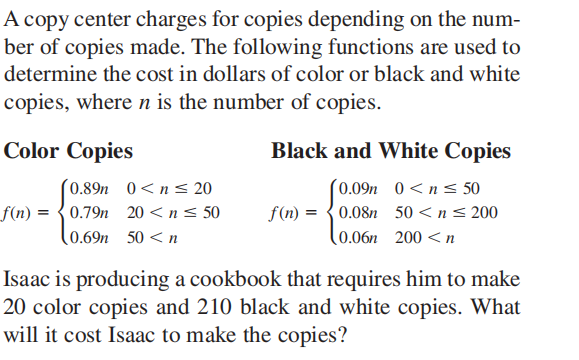### ¿Todavía tienes preguntas de matemáticas?

Pregunte a nuestros tutores expertos
Algebra
PreguntaA copy center charges for copies depending on the number of copies made. The following functions are used to determine the cost in dollars of color or black and white copies, where $$n$$ is the number of copies.

Color Copies

$$f ( n ) = \left\{ \begin{array} { l l } { 0.89 n } & { 0 < n \leq 20 } \\ { 0.79 n } & { 20 < n \leq 50 } \\ { 0.69 n } & { 50 < n } \end{array} \right.$$

Black and White Copies

$$f ( n ) = \left\{ \begin{array} { l l } { 0.09 n } & { 0 < n \leq 50 } \\ { 0.08 n } & { 50 < n \leq 200 } \\ { 0.06 n } & { 200 < n } \end{array} \right.$$

Isaac is producing a cookbook that requires him to make $$20$$ color copies and $$210$$ black and white copies. What will it cost Isaac to make the copies?

$$\ 30.40$$